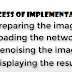Denoise Image Using Deep Convolutional Neural Networks. The Deep Convolutional Neural Networks (DnCNN) is the class of deep neural networks that performs the visual analysis of the image. The image denoising is a classical research field of image processing but still an active topic. The emergence of Deep Convolutional Neural Networks created a boom in this field due to its various merits mentioned below in the bullet points.
• It saves time and affords.
• The pre-trained denoising networks are very well trained.
• It generates excellent denoising results.
If you want to understand Image Denoising Using Deep Convolutional Neural Networks, its process of implementation, and implementation code with brief explanation, then you are on the correct page.

#### IMPLEMENTATION CODE

ntwrk = denoisingNetwork('DnCNN');
`IJK = imread('IMAGE_NAME_WITH_ITS_FORMAT');`
`noisyIMAGE = imnoise(IJK,'gaussian',0,0.02);`
`montage({IJK,noisyIMAGE})`
`title('Original Image and Degraded Image in the left and right respectively')`
`denoisedIMAGE = denoiseImage(noisyIMAGE,ntwrk);`
`imshow(denoisedIMAGE)`
`title('Denoised Image')`

### Brief Explanation

• Collect, load, and process the image.
// The standard cameraman image dataset is loaded using imread() function an stored in I.

noisyI = imnoise(I,'gaussian',0,0.01);
// Now Gaussian noise is added to the I image using imnoise() function and stored in noisyI. The mean of the noise is 0. The variance (density) of the noise is 0.01.

montage({I,noisyI})
title('Original Image (Left) and Noisy Image (Right)'
// Show the original and noisy image.

• Load the pre-trained denoising network.
net = denoisingNetwork('DnCNN');
// Now load the pretrained denoising convolutional neural network, i.e. 'DnCNN' using denoisingNetwork() function and store it in 'net' variable.
• Passing the noisy image to the pre-trained denoising network and getting the denoised image.
denoisedI = denoiseImage(noisyI,net);
// Now passing the noisy image i.e. 'noisyI' to the pre-trained denoising network i.e. 'net' and getting the denoised image i.e. 'denoisedI'. This is done by using denoiseImage() function.
// Let say when noisy image 'A' is passed pre-trained denoising network, then we get the residual image 'B'. [Denoised image = A - B]

• Showing the result.

imshow(denoisedI)
title('Denoised Image')
// The final denoised image is showed using imshow( function) and titled as Denoised Image.

References:

1. https://in.mathworks.com/help/images/ref/denoiseimage.html
2. https://in.mathworks.com/help/images/deep-learning.html?s_tid=CRUX_lftnav
3. https://in.mathworks.com/help/images/ref/denoisingnetwork.html
4. Kai Zhang, Wangmeng Zuo, Senior Member, IEEE, Yunjin Chen, Deyu Meng, and Lei Zhang, Senior Member, IEEE, "Beyond a Gaussian Denoiser: Residual Learning of Deep CNN for Image Denoising", IEEE TRANSACTIONS ON IMAGE PROCESSING, VOL. 26, NO. 7, JULY 2017.
Denoise Image Using Deep Convolutional Neural Networks | Image Denoising | Deep Learning for Image Processing | MATLAB Deep Learning Toolbox™Reviewed by IPR on May 22, 2020 Rating: 5
1.